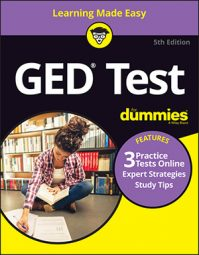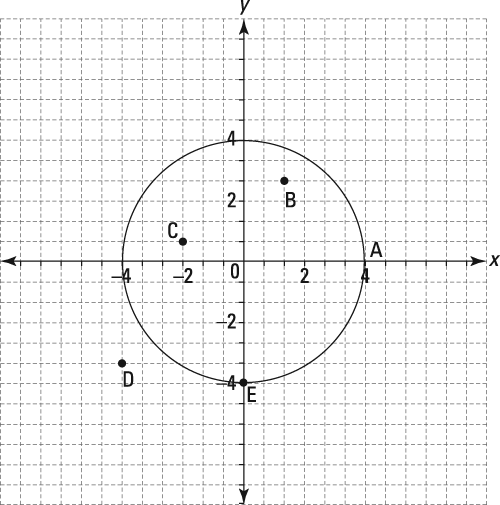##### GED Test 2022 / 2023 For Dummies with Online PracticeIf you enjoy locating points on the coordinate plane, then some questions on the GED Math test will have you smiling. Coordinate plane questions won’t just test your ability to work with x and y coordinates, though; as you’ll see in the following practice questions, you’ll also need to brush up on your geometry skills.

## Practice questions

1. A rectangle 5 units long and 4 units high is represented on a graph. If three of the corners are placed at (3, 2), (3, –2), and (–2, 2), where should the fourth corner be placed?A. (–2, 2) B. (2, –2) C. (–2, –2) D. (2, 2)
2. A circle is drawn with its center at the origin and a diameter of 8 units. Where will the circumference intersect the negative y-axis? Circle this point on the graph.1. The correct answer is Choice (C).This question tests your skills in geometry by asking you to visualize a graph of an object. Because the object is a rectangle, the opposite sides are equal in length and are parallel, the fourth corner will be 2 units to the left of the y-axis, giving it an x-coordinate of –2, and 2 units below the x-axis, giving it a y-coordinate of –2. Therefore, the point would be (–2, –2).

The x-coordinate is the distance from the y-axis, and the y-coordinate is the distance from the x-axis.

2. The correct answer is Point E (0, –4).If the center is at the origin and the diameter (which is twice the radius) is 8 units, the circle will intersect each of the axes (that’s the plural of axis) at a distance of 4 units from it. Therefore, it intersects the negative y-axis at (0, –4) or Point E.